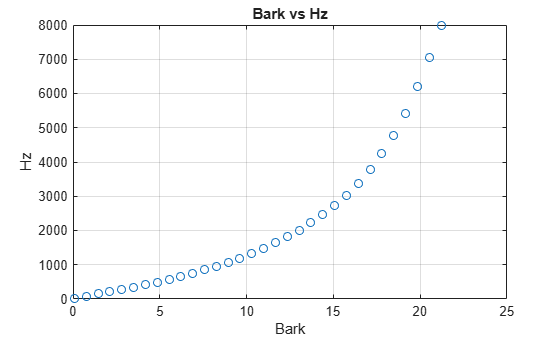bark2hz

Convert from Bark scale to hertz

Description

example

hz = bark2hz(bark) converts values on the Bark frequency scale to values in hertz.

Examples

collapse all

Set two bounding frequencies in Hz and then convert them to the Bark scale.

b = hz2bark([20,8000]);

Generate a row vector of 32 values uniformly spaced on the Bark scale.

barkVect = linspace(b(1),b(2),32);

Convert the row vector of values into equivalent frequencies in Hz.

hzVect = bark2hz(barkVect);

Plot the two vectors for comparison. As Bark values increase linearly, Hz values increase exponentially.

plot(barkVect,hzVect,'o')
title('Bark vs Hz')
xlabel('Bark')
ylabel('Hz')
grid onInput Arguments

collapse all

Input frequency on the Bark scale, specified as a scalar, vector, matrix, or multidimensional array.

Data Types: single | double

Output Arguments

collapse all

Output frequency in Hz, returned as a scalar, vector, matrix, or multidimensional array the same size as bark.

Data Types: single | double

Algorithms

The frequency conversion from the Bark scale to Hz uses the following formula:

$\begin{array}{l}if:bark<2\to bark=\frac{\left(bark-0.3\right)}{0.85}\\ if:bark>20.1\to bark=\frac{\left(bark+4.422\right)}{1.22}\\ hz=1960\left(\frac{bark+0.53}{26.28-bark}\right)\end{array}$

The Bark value correction occurs before the conversion from the Bark scale to Hz.

 Traunmüller, Hartmut. "Analytical Expressions for the Tonotopic Sensory Scale." Journal of the Acoustical Society of America. Vol. 88, Issue 1, 1990, pp. 97–100.

Audio Toolbox Documentation